# Half Adder Circuit and its Construction

Published  June 25, 2018   0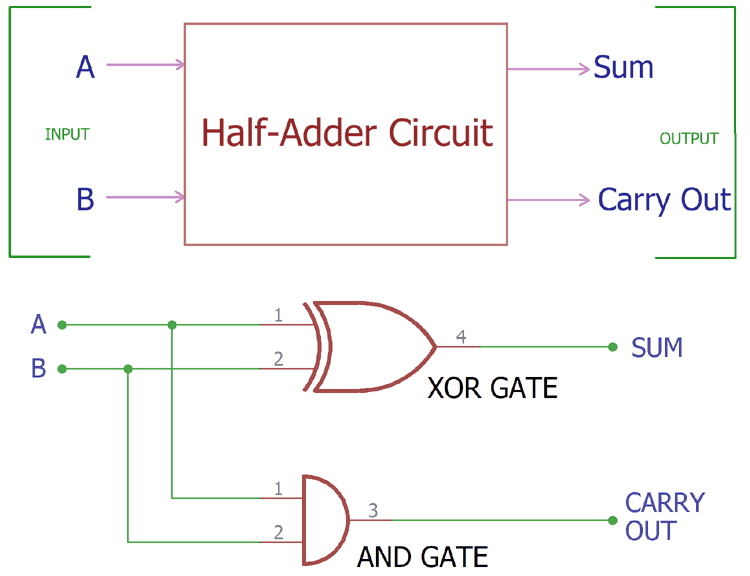Computer uses binary numbers 0 and 1. An adder circuit uses these binary numbers and calculates the addition. A binary adder circuit can be made using EX-OR and AND gates. The summation output provides two elements, first one is the SUM and second one is the Carry Out.

When we use arithmetic summation process in our base 10 mathematics, like adding two numbers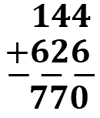We add each column from right to left and if the addition greater than or equal to 10, we use carry. In the first addition 6+4 is 10. We wrote 0 and carry the 1 to the next column. So, each value has a weighted value based on its column position.

In case of binary number addition the process is same. Instead of the two denary numbers here binary numbers are used. In binary, we only get two numbers either 1 or 0. These two numbers can represent SUM or CARRY or both. As in binary number system, 1 is the largest digit, we only produce carry when the addition is equal or greater than 1 + 1 and due to this, carry bit will be passed over next column for addition.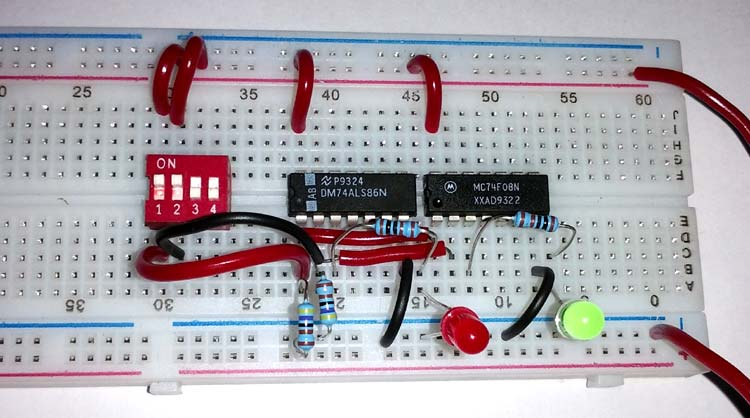Below is the block diagram of a Half-Adder, which requires only two inputs and provide two outputs.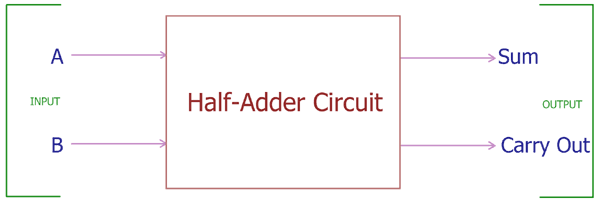Let’s see possible binary addition of two bits,

 1st Bit or Digit 2nd Bit or Digit Sum of the total< Carry 0 0 0 0 1 0 1 0 0 1 1 0 1 1 0 1

The first digit, we can denote as A and the second digit we can denote as B, are added together and we can see the summation result and carry bit. In the first three row 0 + 0, 0 + 1 or 1+ 0 the addition is 0 or 1 but there is no carry bit, But in the last row we added 1 + 1  and it is produce a carry bit of 1 along with result 0.

So, if we see the operation of an adder circuit, we need only two inputs and it will produce two outputs, one is addition result, denoted as SUM and other one is CARRY OUT bit.

### Construction of Half Adder Circuit:

We have seen the Block Diagram of Half Adder circuit above with two inputs A,B and two outputs- Sum, Carry Out.  We can make this circuit using two basic gates

1. 2-input Exclusive-OR Gate or Ex-OR Gate
2. 2-input AND Gate.

2-input Exclusive-OR Gate or Ex-OR Gate

The Ex-OR gate is used to produce the SUM bit and AND Gate produce the carry bit of the same input A and B.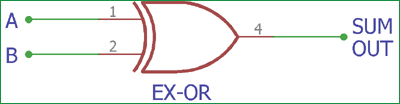This is the symbol of two inputs EX-OR gate. A, and B is the two binary input and SUMOUT is the final output after adding two numbers.

The truth table of EX-OR gate is

 Input A Input B SUM OUT 0 0 0 0 1 1 1 0 1 1 1 0

In the above table we can see the total sum output of the EX-OR gate. When any one of the bits A and B is 1 the output of the gate becomes 1.  On the two other cases when both inputs are 0 or 1 the Ex-OR gate produce 0 outputs. Learn more about EX-OR gate here.

2-input AND Gate:

X-OR gate only provides the sum and unable to provide carry bit on 1 + 1, we need another gate for Carry. AND gate is perfectly fits in this application.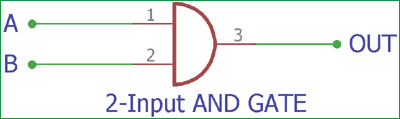This is the basic circuit of two input AND gate. Same as like EX-OR gate it has two inputs. If we provide A and B bit in the input it will produce an Output.

The output is depends on the AND gate truth table-

 Input A Input B Carry Output 0 0 0 0 1 0 1 0 0 1 1 1

In the above, the truth table of AND gate is shown where it will only produce the output when both inputs are 1, Otherwise it will not provide an output if both inputs are 0 or any of the inputs is 1. Learn more about AND gate here.

So the Half-Adder logical circuit can be made by combining this two gates and providing the same input in both gates.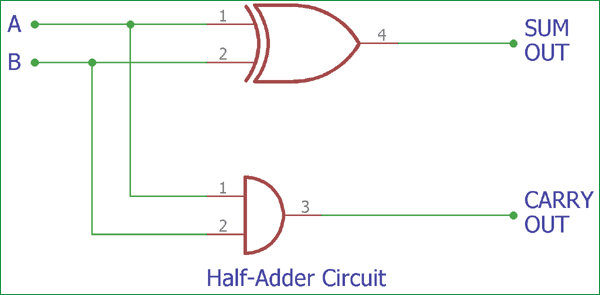This is the construction of Half-Adder circuit, as we can see two gates are combined and the same input A and B are provided in both gates and we get the SUM output across EX-OR gate and the Carry Out bit across AND gate.

The Boolean expression of Half Adder circuit is-

```SUM = A XOR B (A+B)
CARRY = A AND B (A.B)```

Truth table of Half-Adder circuit is as follows-

 Input A Input B SUM (XOR out) CARRY (AND out) 0 0 0 0 1 0 1 0 0 1 1 0 1 1 0 1

### Practical Demonstration of Half Adder Circuit:

We can make the circuit in real on breadboard to understand it clearly. For this we used two widely used XOR and AND chip from 74 series 74LS86 and 74LS08.

Both are gate ICs. 74LS86 has four XOR gates inside the chip and 74LS08 has four AND gates inside it. These two ICs are widely available and we will make Half-Adder circuit using this two.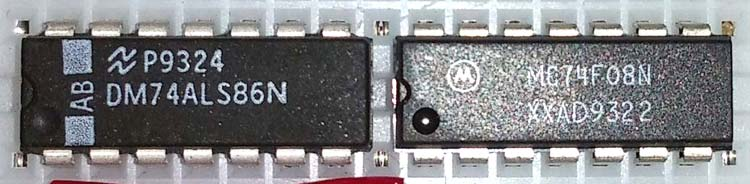Below is the Pin Diagram for both the ICs: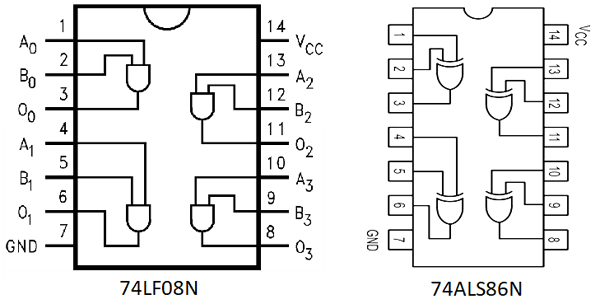Circuit Diagram to use these two ICs as a half-adder circuit-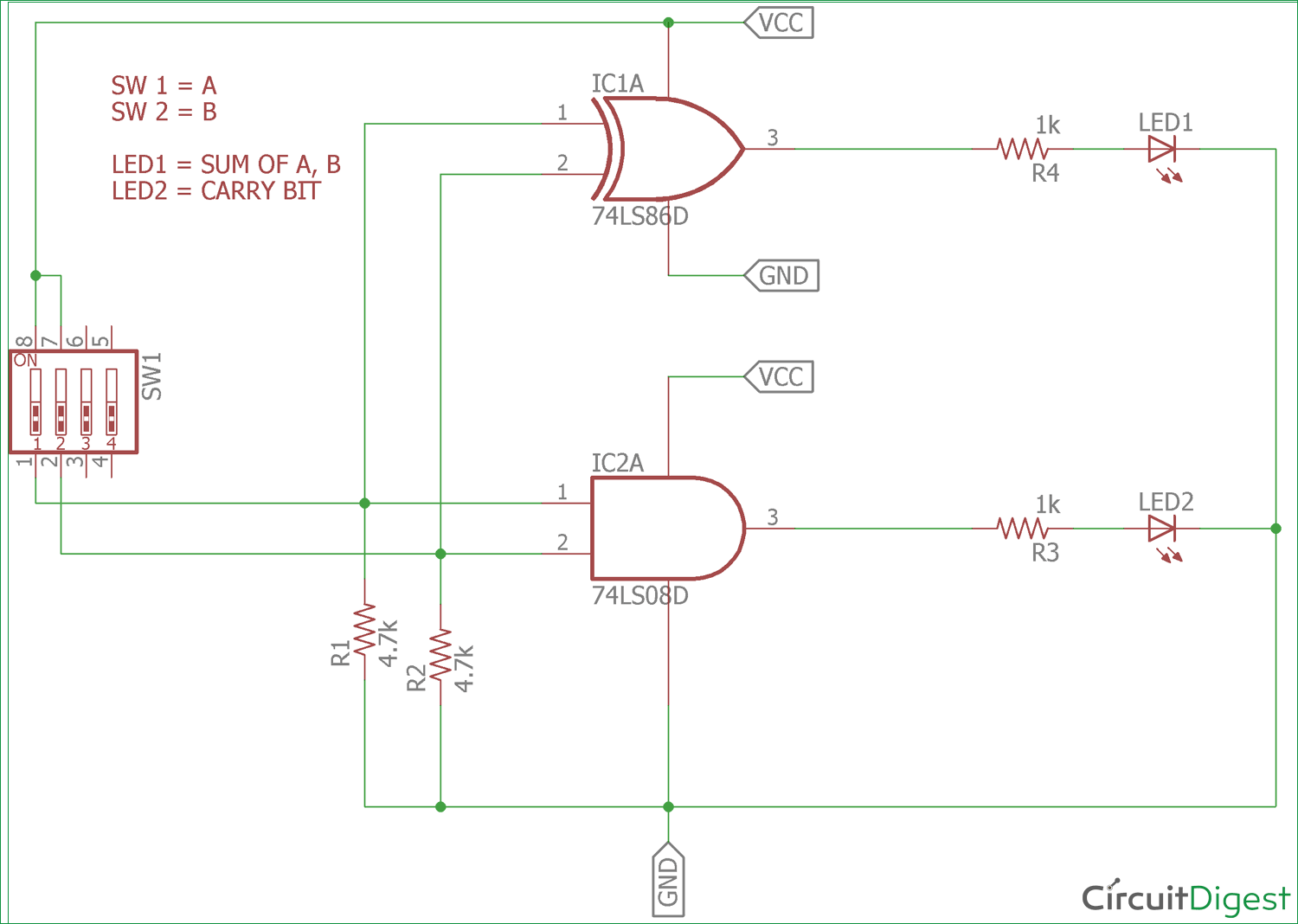We constructed the circuit in breadboard and observed the output.In the above circuit diagram one of the XOR gate from 74LS86 is used and also one of the AND gate from 74LS08 is used. Pin 1 and 2 of 74LS86 is the input of the gate and pin 3 is the output of the gate, on the other side pin 1 and 2 of 74LS08 is the input of the AND gate and pin 3 is the output of the gate. Pin No 7 of both ICs is connected to GND and 14th pin of the both ICs is connected to VCC. In our case the VCC is 5v. We added two leds to identify the output.  When the output is 1, the LED will glow.

We added DIP switch in the circuit to provide input on the gates, for the bit 1 we are providing 5V as input and for 0 we are providing GND through 4.7k resistor. 4.7k resistor is used to provide 0 inputs when the switch is in off state.

Demonstration Video is given below.

Half Adder circuit is used for bit addition and logical output related operations in computers. Also, it has a major disadvantage that we cannot provide carry bit in the circuit with A and B input. Due to this limitation the full adder circuit is constructed.